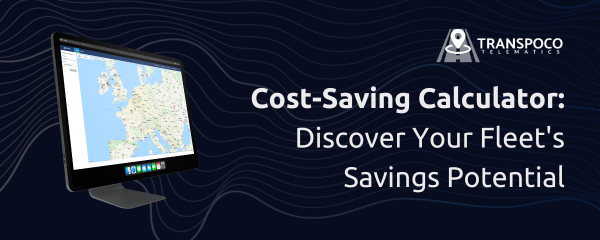Knowledge Base

# How is Fuel Consumption Calculated?

## Formula and example of how to calculate fuel in L/100 km with fuel transaction data

Ever wondered how fuel consumption is precisely calculated? It's a crucial metric for understanding the efficiency of your vehicle. Let's delve into the formula and provide an illustrative example of calculating fuel consumption in litres per 100 kilometers (L/100 km) using fuel transaction data.

Formula: Fuel Consumption (L/100 km) = (Litres Used X 100) ÷ Kilometers Traveled

This formula is widely recognized as the standard for measuring fuel consumption. It allows you to assess how efficiently your vehicle uses fuel.

To perform this calculation, you'll need the following data:

• Odometer reading at the 1st fuel fill (e.g., 1000 km)
• Odometer reading at the 2nd fuel fill (e.g., 1500 km)
• The amount of fuel added to fill the tank to the maximum at the 2nd fill (Litres Used, e.g., 65 litres)

Illustrative Example: Let's consider a scenario where a car consumes 65 litres of fuel to travel a distance of 500 kilometers. To calculate its fuel consumption in L/100 km:

(65 X 100) ÷ 500 = 13 Litres/100 km

In this case, the car's fuel consumption rate is 13 L/100 km, indicating that it consumes 13 litres of fuel to cover a distance of 100 kilometers. This measurement provides valuable insights into the efficiency of the vehicle's fuel usage and is a valuable tool for both individuals and fleet managers looking to optimize fuel efficiency.